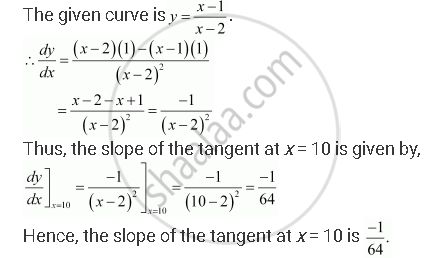Share

# Find the Slope of the Tangent to the Curve Y = (X -1)/(X - 2), X != 2 At X = 10. - CBSE (Commerce) Class 12 - Mathematics

#### Question

Find the slope of the tangent to the curve y = (x -1)/(x - 2), x != 2 at x = 10.

#### SolutionIs there an error in this question or solution?

#### APPEARS IN

NCERT Solution for Mathematics Textbook for Class 12 (2018 to Current)
Chapter 6: Application of Derivatives
Q: 2 | Page no. 211

#### Video TutorialsVIEW ALL 

Solution Find the Slope of the Tangent to the Curve Y = (X -1)/(X - 2), X != 2 At X = 10. Concept: Tangents and Normals.
S